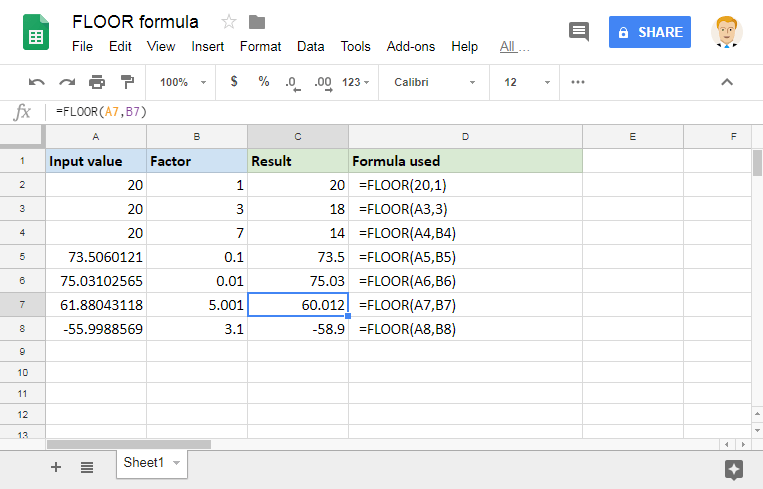# How to use the FLOOR formula in Google SheetsWhile there are infinite rational numbers in mathematics, we don’t usually deal with all of them. That’s because, in real life, it isn’t even feasible. Consider currency for instance. We may make many money transaction calculations – interest, exchange rates, etc. For the purposes of this example, let’s say we arrived at \$21.835. If we were to transact that in reality, we may have to round it off to the nearest available currency denomination. Because it is unlikely that we have physical denominations available to pay that amount in entirety. And more often, we round it down to the lowest possible multiple-denomination value. For instance, if we had \$10 bills, we would round the value \$21.835 to \$20. Or if we had \$1 bills, then we’d round it to \$21. The FLOOR formula in Google Sheets helps us with the similar scenarios while handling numbers on spreadsheets.

### Syntax

FLOOR(value, [factor])

• value – is the value which we are trying to round down to the nearest integer multiple of the factor.
• factor – [OPTIONAL – 1 by default] – is the number to whose multiples, the formula rounds the value to.

Please note that the factor may not be equal to 0, and is usually a positive value.

### Usage: FLOOR formula in Google Sheets

The formula syntax isn’t that complicated. But, it can be a little tricky to understand its behavior. So let us make use of a few examples to clear out any doubts. Consider the following screenshot.Let us consider the first example. If we look at the multiples of factor value 1, they are 1, 2, 3, …18, 19, 20, 21, … etc. Since 20 is the nearest available factor multiple, the formula returned 20 as output.

Similarly, in the second example, the factor multiples are 3, 6, 9, 12, 15, 18, 21, 24, … etc. Clearly, the value 20 lies between the multiples 18 and 21. Since the formula considers the nearest lower value (or the FLOOR value), it results in 18 and not 21.

Same is the case with the third example. Among the multiples 7, 14, 21, 28, … etc. the FLOOR of the value 20 is 14. We can also extend this to even rational factors, as demonstrated in the final four examples. Please note that we can also use negative values for the first input parameter (final example).